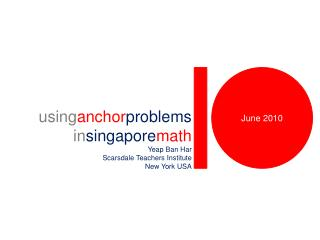DownloadDownload PresentationJune 2010

# June 2010

Télécharger la présentation## June 2010

- - - - - - - - - - - - - - - - - - - - - - - - - - - E N D - - - - - - - - - - - - - - - - - - - - - - - - - - -
##### Presentation Transcript

1. June 2010 usinganchorproblems insingaporemath Yeap Ban Har Scarsdale Teachers Institute New York USA

2. Problem 1 Arrange cards numbered 1 to 10 so that the trick shown by the instructor can be done. In this problems, students get to talk about the positions of the cards using ordinal numbers. They get to use ordinal numbers in two contexts – third card from the left and third card from the top. The problem itself is too tedious to describe using words. Just come for the institute next time round!

3. Teachers solved the problems in different ways.

4. The above is the solution. What if the cards used are numbered 1 to 9? 1 to 8? 1 to 7? 1 to 6? 1 to 5? 1 to 4?

5. Problem 2 This is a game for two players. The game can start with any number of paper clips, say, 24. The rule is that a player removes exactly 1 or 2 clips when it is his / her turn. The winner is the player who removes the last lot of paper clips. Find out a way to win the game.

6. It turns out that the winning strategy is to leave your opponent with a multiple of three. What if the rule is changed to removing exactly 1, 2 or 3 clips? The winning strategy is to leave your opponent with a multiple of four. Did you notice a pattern? Problem 2 In this anchor problem and its variant(s), the concept of multiples emerges. Anchor problem does that – to emerge a mathematical concept.

7. Problem 3 The initial textbook problem was changed into the second one. Anchor problems and its variants allow students to encounter a range of problems with mathematical variability (Dienes). In this case students encounter both arithmetic and algebraic problems, and also problems with continuous as well as discrete quantities. Although both problems involve the same bar models, the mathematics involved are different.

8. 273 Najib Pascal 297 Goggle Gittar

9. Problem 4 • Mrs Liu spent 1/5 of her monthly salary on a handbag,  of the remainder on a vacuum cleaner and saved the rest. She saved \$1890. Find her monthly salary. • = half • = three-eighths • = four-sevenths The anchor problem was varied systematically so that students get to learn different skills in bar modeling. Notice the different bar modeling skills needed to solve the problem when  is changed from half to three-eighths to four-sevenths. This problem is taken from a Singapore school’s mock examination to prepare students for the national examination at the end of grade six.

10. Three methods to solve the third problem.

11. Problem 5 Think of a 4-digit number, say, 1104. Jumble the digits up to form a different number, say, 0411. Fin d the difference between the two numbers (1104 – 411). Write the difference on a piece of paper. Circle any digit. Tell me the digits you did not circle and I will tell you the one you circled.

12. How can you tell what the circled number is given the numbers which are not circled?

13. Problem 6 Piece the pieces together so that two adjacent values are equal. Initially students can be asked to simply piece any two pieces together. Later they can be asked to form a single ‘snaking’ figure. Finally they can be challenge to form a square.

14. Problem 7 Place digits 0 to 9 in the five spaces to make a correct multiplication sentence – no repetition. 2-digit number multiplied by 1-digit number to give a 2-digit product. x

15. Why are there so few odd products?

16. Summary anchorproblems

17. Roles • To emerge the idea of multiples • To provide opportunities to use ordinal numbers in varied situations • To provide opportunity to solve arithmetic and algebraic problems that involve continuous and discrete quantities • To provide opportunity to learn different skills in using bar models • To provide opportunity to do drill-and-practice while

18. Participants working through anchor problems they created. workshopactivity

19. References theoriesandmodels

20. Polya Polya Problem-Solving Stages • Understand • Plan • Do • Look Back

21. Newman Newman Difficulties in Word Problem Solving • Read • Comprehend • Know Strategies • Transform • Do Procedures • Interpret Answers

22. Dienes Dienes • Principle of Variability • Mathematical Variability • Perceptual Variability

23. Krutetskii Krutetskii • The ability to pose a ‘natural’ question as a form of mathematical ability Krutetski (1976). The psychology of mathematical abilities in school children. Chicago, IL: University of Chicago.

24. Big Ideas Big Ideas • Visualization • Patterning • Number Sense • Metacognition • Communication

25. Beliefs Interest Appreciation Confidence Perseverance Monitoring of one’s own thinking Self-regulation of learning Attitudes Metacognition Numerical calculation Algebraic manipulation Spatial visualization Data analysis Measurement Use of mathematical tools Estimation Mathematical Problem Solving Reasoning, communication & connections Thinking skills & heuristics Application & modelling Skills Processes Concepts Numerical Algebraic Geometrical Statistical Probabilistic Analytical Mathematics Curriculum Framework